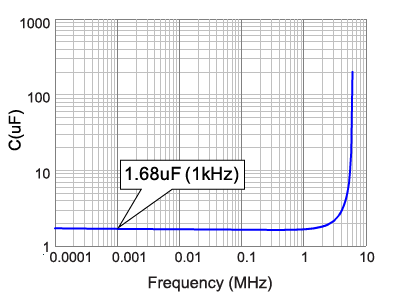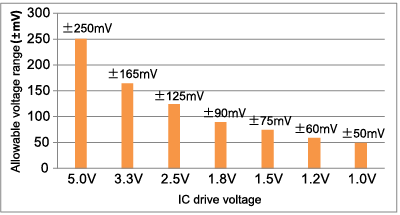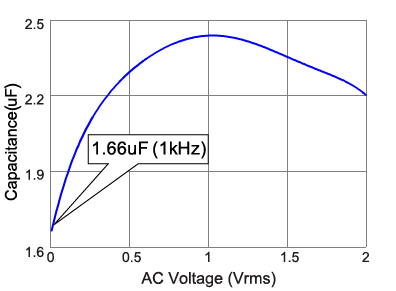# Ceramic Capacitors FAQQThe capacitance-frequency characteristics displayed by SimSurfing differ from the nominal capacitance. What is the reason for this?

A
This is thought to be due to a difference in the measurement conditions.

In the capacitance-frequency characteristics (C-f characteristics) of multilayer ceramic capacitors that can be checked using the design support tool SimSurfing, the displayed capacitance may be lower than the nominal capacitance. For example, the nominal capacitance of the GRM155B30J225KE95 is 2.2 uF, but the C-f characteristics shown in Figure 1 indicate the lower value of 1.68 uF. This is because the measurement voltage applied to capacitors when measuring the frequency characteristics is set to a lower value compared to the measurement conditions for the nominal capacitance.
The reason why the C-f characteristics are lower than the nominal capacitance is explained below together with the background.Figure 1: Capacitance-frequency characteristics (GRM155B30J225KE95)

### Characteristics required of capacitors as IC drive voltages become lower

IC drive voltages are continuing to decrease in order to support higher IC integration, higher operating frequencies and reduced power consumption. IC drive voltages that were 5.0 V in the early 1990s have been progressively lowered to 3.3 V, 2.5 V, 1.8 V, 1.5 V, 1.2 V and now 1.0 V.
The allowable voltage fluctuation has also decreased together with the IC drive voltage. For example, given an accuracy tolerance of ±5%, voltage fluctuation of ±0.25 V between 4.75 V and 5.25 V can be tolerated at 5.0 V, but voltage fluctuation of only ±0.05 V can be tolerated at 1.0 V (Figure 2).
In response to these severe voltage requirements, various countermeasures are taken to suppress voltage fluctuation, such as the use of Point of Load (POL) power supply or the use of multilayer ceramic capacitors as smoothing capacitors and decoupling capacitors. In these cases, AC voltage (ripple or fluctuation) of several tens mV or less in the IC drive voltage is applied to the capacitor, making performance under this condition an important capacitor characteristic.Figure 2:  IC drive voltages and allowable ranges (accuracy tolerance 5%)

### Frequency characteristics and nominal capacitance measurement conditions

As previously described, IC drive voltages are becoming lower with the result that multilayer ceramic capacitors are increasingly used under conditions where voltage of several tens mV is applied. This means that data measured at low signal voltages is needed in order to indicate the capacitor characteristics. Therefore, the measurement conditions for the frequency characteristics data released by SimSurfing are set so that the measurement voltage applied to the capacitor is several tens mV or less.
High dielectric constant-type multilayer ceramic capacitors have AC voltage dependence characteristics, and many exhibit a tendency for the capacitance to decrease together with the AC voltage. Therefore, the frequency characteristics data is the result of measurement at low signal voltages, which produces lower values compared to the nominal capacitance.
Figure 3 shows the AC voltage characteristics of the GRM155B30J225KE95. Here, the capacitance value at AC 10 mVrms is 1.66 uF, which approximately matches the capacitance-frequency characteristics shown in Figure 1.Figure 3: AC voltage characteristics (GRM155B30J225KE95)

#### Related FAQ

We appreciate your cooperation with the FAQ improvement questionnaire.